# Floquet calibration: Example and benchmark

This tutorial shows a detailed example and benchmark of Floquet calibration, a calibration technique introduced in the Calibration: Overview and API tutorial.

## Setup

try:
import cirq
except ImportError:
print("installing cirq...")
!pip install --quiet cirq --pre
print("installed cirq.")

from typing import Iterable, List, Optional, Sequence

import matplotlib.pyplot as plt
import numpy as np
import os

import cirq
import cirq_google as cg  # Contains the Floquet calibration tools.


No project_id provided and environment variable GOOGLE_CLOUD_PROJECT not set.
Using a noisy simulator.


## Defining the circuit

We run Floquet calibration on a circuit which models the evolution of a single fermionic particle on 5 sites, realizing the Hamiltonian:

$H=\sum_{m=0}^{L-1} J(\sigma_{m}^{+} \sigma_{m+1}^{-} + \sigma_{m}^{+} \sigma_{m+1}^{-}),$

where $$\sigma_{m}^{+}$$ ($$\sigma_{m}^{-}$$) are the raising (lowering) operators, and the single term describes the kinetic energy related to hopping from one site to the other. This quirk circuit shows the evolution of the charge density.

This simulation can be looked at as a highly simplified version of the paper from our group, Observation of separated dynamics of charge and spin in the Fermi-Hubbard model. We model only a single fermion in the non-interacting case (with $$U=0$$). For a single particle, the parasitic controlled phase does not impact the evolution, and we can use a single chain that one can think about it as being in either up or down spin states. The parameter $$\theta$$ for $$K(\theta)$$ is fixed to $$\pi/4$$. To smooth out the inhomogeneities of the quantum chip, we are using the technique of averaging over multiple qubit configurations from this paper. The difference is that we pick a line that we segment and run the same circuit in parallel on each segment corresponding to a different qubit configuration. We measure the charge density at each site index (qubit) by averaging the Z densities (see Fig 2.a for comparison).

The physics of this problem for a closed chain (here we use an open chain) has been studied in Accurately computing electronic properties of materials using eigenenergies as well without the complex hopping term, hence we use no Z rotations between the $$\sqrt{\text{iSWAP} }$$ gates. This paper also describes the Floquet calibration fundamentals in Appendix A.

First we use the function cirq_google.line_on_device to return a line of qubits of a specified length.

line = cg.line_on_device(device_sampler.device, line_length)
print(line)

(0, 5)━━(0, 6)
┃
(1, 6)━━(1, 7)
┃
(2, 6)  (2, 7)━━(2, 8)
┃               ┃
(3, 6)━━(3, 7)  (3, 8)━━(3, 9)
┃               ┃
(4, 7)━━(4, 8)  (4, 9)━━(4, 10)
┃               ┃
(5, 8)━━(5, 9)  (5, 10)
┃       ┃
(6, 9)━━(6, 10)


This line is now broken up into a number of segments of a specified length (number of qubits).

segment_length = 5
segments = [line[i: i + segment_length]
for i in range(0, line_length - segment_length + 1, segment_length)]


For example, the first segment consists of the following qubits.

print(*segments)

(0, 5) (0, 6) (1, 6) (1, 7) (2, 7)


We now implement a number of Trotter steps on each segment in parallel. The middle qubit on each segment is put into the $$|1\rangle$$ state, then each Trotter step consists of staggered $$\sqrt{\text{iSWAP} }$$ gates. All qubits are measured in the $$Z$$ basis at the end of the circuit.

For convenience, this code is wrapped in a function.

sqrt_iswap = cirq.ISWAP ** 0.5

def create_linear_chain_segment(
segment: Sequence[cirq.Qid],
num_trotter_steps: int,
) -> cirq.Circuit:
"""Returns a linear chain circuit on one segment."""
circuit = cirq.Circuit(cirq.X.on(segment[len(segment) // 2]))

# Trotter steps.
for step in range(num_trotter_steps):
offset = step % 2
circuit += cirq.Moment(
[sqrt_iswap.on(a, b) for a, b in zip(segment[offset::2],
segment[offset + 1::2])])
return circuit

def create_linear_chain_circuit(
segments: Sequence[Sequence[cirq.Qid]],
num_trotter_steps: int,
) -> cirq.Circuit:
"""Returns a linear chain circuit to demonstrate Floquet calibration on."""
circuit_segments = [create_linear_chain_segment(segment, num_trotter_steps) for segment in segments]
circuit = cirq.Circuit.zip(*circuit_segments)
return circuit + cirq.measure(*sum(segments, ()), key='z')


As an example, we show this circuit on the first segment of the line from above.

"""Example of the linear chain circuit on one segment of the line."""
num_trotter_steps = 20

circuit_on_segment = create_linear_chain_circuit(
segments=[segments],
num_trotter_steps=num_trotter_steps,
)
print(circuit_on_segment.to_text_diagram(qubit_order=segments))

(0, 5): ───────iSwap───────────────────iSwap───────────────────iSwap───────────────────iSwap───────────────────iSwap───────────────────iSwap───────────────────iSwap───────────────────iSwap───────────────────iSwap───────────────────iSwap───────────────────M('z')───
│                       │                       │                       │                       │                       │                       │                       │                       │                       │                       │
(0, 6): ───────iSwap^0.5───iSwap───────iSwap^0.5───iSwap───────iSwap^0.5───iSwap───────iSwap^0.5───iSwap───────iSwap^0.5───iSwap───────iSwap^0.5───iSwap───────iSwap^0.5───iSwap───────iSwap^0.5───iSwap───────iSwap^0.5───iSwap───────iSwap^0.5───iSwap───────M────────
│                       │                       │                       │                       │                       │                       │                       │                       │                       │           │
(1, 6): ───X───iSwap───────iSwap^0.5───iSwap───────iSwap^0.5───iSwap───────iSwap^0.5───iSwap───────iSwap^0.5───iSwap───────iSwap^0.5───iSwap───────iSwap^0.5───iSwap───────iSwap^0.5───iSwap───────iSwap^0.5───iSwap───────iSwap^0.5───iSwap───────iSwap^0.5───M────────
│                       │                       │                       │                       │                       │                       │                       │                       │                       │                       │
(1, 7): ───────iSwap^0.5───iSwap───────iSwap^0.5───iSwap───────iSwap^0.5───iSwap───────iSwap^0.5───iSwap───────iSwap^0.5───iSwap───────iSwap^0.5───iSwap───────iSwap^0.5───iSwap───────iSwap^0.5───iSwap───────iSwap^0.5───iSwap───────iSwap^0.5───iSwap───────M────────
│                       │                       │                       │                       │                       │                       │                       │                       │                       │           │
(2, 7): ───────────────────iSwap^0.5───────────────iSwap^0.5───────────────iSwap^0.5───────────────iSwap^0.5───────────────iSwap^0.5───────────────iSwap^0.5───────────────iSwap^0.5───────────────iSwap^0.5───────────────iSwap^0.5───────────────iSwap^0.5───M────────


The circuit we will use for Floquet calibration is this same pattern repeated on all segments of the line.

"""Circuit used to demonstrate Floquet calibration."""
circuit = create_linear_chain_circuit(
segments=segments,
num_trotter_steps=num_trotter_steps
)


## Execution on a simulator

To establish a "ground truth," we first simulate a segment on a noiseless simulator.

"""Simulate one segment on a simulator."""
nreps = 20_000
sim_result = cirq.Simulator().run(circuit_on_segment, repetitions=nreps)


## Execution on the processor without Floquet calibration

We now execute the full circuit on a processor without using Floquet calibration.

"""Execute the full circuit on a processor without Floquet calibration."""
raw_results = device_sampler.sampler.run(circuit, repetitions=nreps)


### Comparing raw results to simulator results

For comparison we will plot densities (average measurement results) on each segment. Such densities are in the interval $$[0, 1]$$ and more accurate results are closer to the simulator results.

To visualize results, we define a few helper functions.

### Helper functions

The next cell defines two functions for returning the density (average measurement results) on a segment or on all segments. We can optionally post-select for measurements with a specific filling (particle number) - i.e., discard measurement results which don't obey this expected particle number.

def z_density_from_measurements(
measurements: np.ndarray,
post_select_filling: Optional[int] = 1
) -> np.ndarray:
"""Returns density for one segment on the line."""
counts = np.sum(measurements, axis=1, dtype=int)

if post_select_filling is not None:
errors = np.abs(counts - post_select_filling)
counts = measurements[errors == 0]

return np.average(counts, axis=0)

def z_densities_from_result(
result: cirq.Result,
segments: Iterable[Sequence[cirq.Qid]],
post_select_filling: Optional[int] = 1
) -> List[np.ndarray]:
"""Returns densities for each segment on the line."""
measurements = result.measurements['z']
z_densities = []

offset = 0
for segment in segments:
z_densities.append(z_density_from_measurements(
measurements[:, offset: offset + len(segment)],
post_select_filling)
)
offset += len(segment)
return z_densities


Now we define functions to plot the densities for the simulator, processor without Floquet calibration, and processor with Floquet calibration (which we will use at the end of this notebook). The first function is for a single segment, and the second function is for all segments.

### Visualizing results

To visualize results, we first extract densities from the measurements.

"""Extract densities from measurement results."""
# Simulator density.
sim_density, = z_densities_from_result(sim_result,[circuit_on_segment])

# Processor densities without Floquet calibration.
raw_densities = z_densities_from_result(raw_results, segments)


We first plot the densities on each segment. Note that the simulator densities ("sim") are repeated on each segment and the lines connecting them are just visual guides.

plot_densities(sim_density, raw_densities, rows=int(np.sqrt(line_length / segment_length)))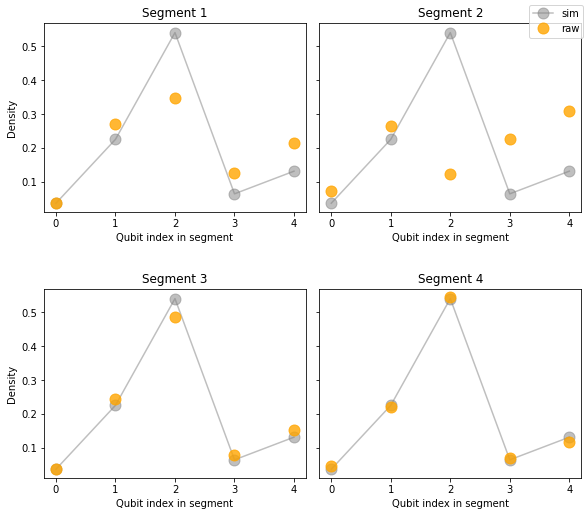We can also look at the average and variance over the segments.

"""Plot mean density and variance over segments."""
raw_avg = np.average(raw_densities, axis=0)
raw_std = np.std(raw_densities, axis=0, ddof=1)

plot_density(
plt.gca(),
sim_density,
raw_density=raw_avg,
raw_errors=raw_std,
title="Average over segments"
)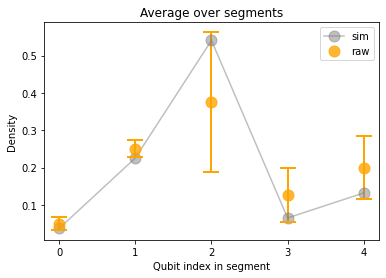In the next section, we will use Floquet calibration to produce better average results. After running the circuit with Floquet calibration, we will use these same visualizations to compare results.

### Execution on the processor with Floquet calibration

There are two equivalent ways to use Floquet calibration which we outline below. A rough estimate for the time required for Floquet calibration is about 16 seconds per 10 qubits, plus 30 seconds of overhead, per calibrated moment.

#### Simple usage

The first way to use Floquet calibration is via the single function call used at the start of this notebook. Here, we describe the remaining returned values in addition to calibrated_circuit.

# (calibrated_circuit, calibrations
#  ) = cg.run_zeta_chi_gamma_compensation_for_moments(
#     circuit,
#     device_sampler.sampler,
# )


The returned calibrated_circuit.circuit can then be run on the engine. The full list of returned arguments is as follows:

• calibrated_circuit.circuit: The input circuit with added $$Z$$ rotations around each $$\sqrt{\text{iSWAP} }$$ gate to compensate for errors.
• calibrated_circuit.moment_to_calibration: Provides an index of the matching characterization (index in calibrations list) for each moment of the calibrated_circuit.circuit, or None if the moment was not characterized (e.g., for a measurement outcome).
• calibrations: List of characterization results for each characterized moment. Each characterization contains angles for each qubit pair.

#### Step-by-step usage

The above function cirq_google.run_zeta_chi_gamma_compensation_for_moments performs the following three steps:

1. Find moments within the circuit that need to be characterized.
2. Characterize them on the engine.
3. Apply corrections to the original circuit.

To find moments that need to be characterized, we can do the following.

"""Step 1: Find moments in the circuit that need to be characterized."""
(characterized_circuit, characterization_requests
) = cg.prepare_characterization_for_moments(
circuit,
options=cg.FloquetPhasedFSimCalibrationOptions(
characterize_theta=False,
characterize_zeta=True,
characterize_chi=False,
characterize_gamma=True,
characterize_phi=False
)
)


The characterization_requests contain information on the operations (gate + qubit pairs) to characterize.

"""Show an example characterization request."""
print(f"Total {len(characterization_requests)} moment(s) to characterize.")

print("\nExample request")
request = characterization_requests
print("Gate:", request.gate)
print("Qubit pairs:", request.pairs)
print("Options: ", request.options)

Total 2 moment(s) to characterize.

Example request
Gate: cirq.FSimGate(theta=0.7853981633974483, phi=0.0)
Qubit pairs: ((cirq.GridQubit(0, 5), cirq.GridQubit(0, 6)), (cirq.GridQubit(1, 6), cirq.GridQubit(1, 7)), (cirq.GridQubit(2, 8), cirq.GridQubit(3, 8)), (cirq.GridQubit(3, 6), cirq.GridQubit(3, 7)), (cirq.GridQubit(3, 9), cirq.GridQubit(4, 9)), (cirq.GridQubit(4, 7), cirq.GridQubit(4, 8)), (cirq.GridQubit(5, 9), cirq.GridQubit(6, 9)), (cirq.GridQubit(5, 10), cirq.GridQubit(6, 10)))
Options:  FloquetPhasedFSimCalibrationOptions(characterize_theta=False, characterize_zeta=True, characterize_chi=False, characterize_gamma=True, characterize_phi=False, readout_error_tolerance=None)


We now characterize them on the engine using cirq_google.run_calibrations.

"""Step 2: Characterize moments on the engine."""
characterizations = cg.run_calibrations(
characterization_requests,
device_sampler.sampler,
max_layers_per_request=1,
)


The characterizations store characterization results for each pair in each moment, for example.

print(f"Total: {len(characterizations)} characterizations.")
print()

(pair, parameters), *_ = characterizations.parameters.items()
print(f"Example pair: {pair}")
print(f"Example parameters: {parameters}")

Total: 2 characterizations.

Example pair: (cirq.GridQubit(0, 5), cirq.GridQubit(0, 6))
Example parameters: PhasedFSimCharacterization(theta=None, zeta=-0.19319183719422314, chi=None, gamma=-0.026983449340713114, phi=None)


Finally, we apply corrections to the original circuit.

"""Step 3: Apply corrections to the circuit to get a calibrated circuit."""
calibrated_circuit = cg.make_zeta_chi_gamma_compensation_for_moments(
characterized_circuit,
characterizations
)


The calibrated circuit can now be run on the processor. We first inspect the calibrated circuit to compare to the original.

print("Portion of calibrated circuit:")
print("\n".join(
calibrated_circuit.circuit.to_text_diagram(qubit_order=line).splitlines()[:9] +
["..."]))

Portion of calibrated circuit:
(0, 5): ────────Rz(-0.474π)───FSim(0.25π, 0)───Rz(0.526π)─────────────────────────────────────────────────Rz(-0.474π)───FSim(0.25π, 0)───Rz(0.526π)─────────────────────────────────────────────────Rz(-0.474π)───FSim(0.25π, 0)───Rz(0.526π)─────────────────────────────────────────────────Rz(-0.474π)───FSim(0.25π, 0)───Rz(0.526π)─────────────────────────────────────────────────Rz(-0.474π)───FSim(0.25π, 0)───Rz(0.526π)─────────────────────────────────────────────────Rz(-0.474π)───FSim(0.25π, 0)───Rz(0.526π)─────────────────────────────────────────────────Rz(-0.474π)───FSim(0.25π, 0)───Rz(0.526π)─────────────────────────────────────────────────Rz(-0.474π)───FSim(0.25π, 0)───Rz(0.526π)─────────────────────────────────────────────────Rz(-0.474π)───FSim(0.25π, 0)───Rz(0.526π)─────────────────────────────────────────────────Rz(-0.474π)───FSim(0.25π, 0)───Rz(0.526π)─────────────────────────────────────────────────M('z')───
│                                                                                         │                                                                                         │                                                                                         │                                                                                         │                                                                                         │                                                                                         │                                                                                         │                                                                                         │                                                                                         │                                                                           │
(0, 6): ────────Rz(0.465π)────FSim(0.25π, 0)───Rz(-0.535π)───Rz(-0.504π)───FSim(0.25π, 0)───Rz(0.496π)────Rz(0.465π)────FSim(0.25π, 0)───Rz(-0.535π)───Rz(-0.504π)───FSim(0.25π, 0)───Rz(0.496π)────Rz(0.465π)────FSim(0.25π, 0)───Rz(-0.535π)───Rz(-0.504π)───FSim(0.25π, 0)───Rz(0.496π)────Rz(0.465π)────FSim(0.25π, 0)───Rz(-0.535π)───Rz(-0.504π)───FSim(0.25π, 0)───Rz(0.496π)────Rz(0.465π)────FSim(0.25π, 0)───Rz(-0.535π)───Rz(-0.504π)───FSim(0.25π, 0)───Rz(0.496π)────Rz(0.465π)────FSim(0.25π, 0)───Rz(-0.535π)───Rz(-0.504π)───FSim(0.25π, 0)───Rz(0.496π)────Rz(0.465π)────FSim(0.25π, 0)───Rz(-0.535π)───Rz(-0.504π)───FSim(0.25π, 0)───Rz(0.496π)────Rz(0.465π)────FSim(0.25π, 0)───Rz(-0.535π)───Rz(-0.504π)───FSim(0.25π, 0)───Rz(0.496π)────Rz(0.465π)────FSim(0.25π, 0)───Rz(-0.535π)───Rz(-0.504π)───FSim(0.25π, 0)───Rz(0.496π)────Rz(0.465π)────FSim(0.25π, 0)───Rz(-0.535π)───Rz(-0.504π)───FSim(0.25π, 0)───Rz(0.496π)────M────────
│                                                                                         │                                                                                         │                                                                                         │                                                                                         │                                                                                         │                                                                                         │                                                                                         │                                                                                         │                                                                                         │                              │
(1, 6): ────X───Rz(-0.503π)───FSim(0.25π, 0)───Rz(0.497π)────Rz(0.538π)────FSim(0.25π, 0)───Rz(-0.462π)───Rz(-0.503π)───FSim(0.25π, 0)───Rz(0.497π)────Rz(0.538π)────FSim(0.25π, 0)───Rz(-0.462π)───Rz(-0.503π)───FSim(0.25π, 0)───Rz(0.497π)────Rz(0.538π)────FSim(0.25π, 0)───Rz(-0.462π)───Rz(-0.503π)───FSim(0.25π, 0)───Rz(0.497π)────Rz(0.538π)────FSim(0.25π, 0)───Rz(-0.462π)───Rz(-0.503π)───FSim(0.25π, 0)───Rz(0.497π)────Rz(0.538π)────FSim(0.25π, 0)───Rz(-0.462π)───Rz(-0.503π)───FSim(0.25π, 0)───Rz(0.497π)────Rz(0.538π)────FSim(0.25π, 0)───Rz(-0.462π)───Rz(-0.503π)───FSim(0.25π, 0)───Rz(0.497π)────Rz(0.538π)────FSim(0.25π, 0)───Rz(-0.462π)───Rz(-0.503π)───FSim(0.25π, 0)───Rz(0.497π)────Rz(0.538π)────FSim(0.25π, 0)───Rz(-0.462π)───Rz(-0.503π)───FSim(0.25π, 0)───Rz(0.497π)────Rz(0.538π)────FSim(0.25π, 0)───Rz(-0.462π)───Rz(-0.503π)───FSim(0.25π, 0)───Rz(0.497π)────Rz(0.538π)────FSim(0.25π, 0)───Rz(-0.462π)───M────────
│                                                                                         │                                                                                         │                                                                                         │                                                                                         │                                                                                         │                                                                                         │                                                                                         │                                                                                         │                                                                                         │                                                                           │
(1, 7): ────────Rz(0.499π)────FSim(0.25π, 0)───Rz(-0.501π)───Rz(-0.466π)───FSim(0.25π, 0)───Rz(0.534π)────Rz(0.499π)────FSim(0.25π, 0)───Rz(-0.501π)───Rz(-0.466π)───FSim(0.25π, 0)───Rz(0.534π)────Rz(0.499π)────FSim(0.25π, 0)───Rz(-0.501π)───Rz(-0.466π)───FSim(0.25π, 0)───Rz(0.534π)────Rz(0.499π)────FSim(0.25π, 0)───Rz(-0.501π)───Rz(-0.466π)───FSim(0.25π, 0)───Rz(0.534π)────Rz(0.499π)────FSim(0.25π, 0)───Rz(-0.501π)───Rz(-0.466π)───FSim(0.25π, 0)───Rz(0.534π)────Rz(0.499π)────FSim(0.25π, 0)───Rz(-0.501π)───Rz(-0.466π)───FSim(0.25π, 0)───Rz(0.534π)────Rz(0.499π)────FSim(0.25π, 0)───Rz(-0.501π)───Rz(-0.466π)───FSim(0.25π, 0)───Rz(0.534π)────Rz(0.499π)────FSim(0.25π, 0)───Rz(-0.501π)───Rz(-0.466π)───FSim(0.25π, 0)───Rz(0.534π)────Rz(0.499π)────FSim(0.25π, 0)───Rz(-0.501π)───Rz(-0.466π)───FSim(0.25π, 0)───Rz(0.534π)────Rz(0.499π)────FSim(0.25π, 0)───Rz(-0.501π)───Rz(-0.466π)───FSim(0.25π, 0)───Rz(0.534π)────M────────
│                                                                                         │                                                                                         │                                                                                         │                                                                                         │                                                                                         │                                                                                         │                                                                                         │                                                                                         │                                                                                         │                              │
(2, 7): ─────────────────────────────────────────────────────Rz(0.507π)────FSim(0.25π, 0)───Rz(-0.493π)────────────────────────────────────────────────Rz(0.507π)────FSim(0.25π, 0)───Rz(-0.493π)────────────────────────────────────────────────Rz(0.507π)────FSim(0.25π, 0)───Rz(-0.493π)────────────────────────────────────────────────Rz(0.507π)────FSim(0.25π, 0)───Rz(-0.493π)────────────────────────────────────────────────Rz(0.507π)────FSim(0.25π, 0)───Rz(-0.493π)────────────────────────────────────────────────Rz(0.507π)────FSim(0.25π, 0)───Rz(-0.493π)────────────────────────────────────────────────Rz(0.507π)────FSim(0.25π, 0)───Rz(-0.493π)────────────────────────────────────────────────Rz(0.507π)────FSim(0.25π, 0)───Rz(-0.493π)────────────────────────────────────────────────Rz(0.507π)────FSim(0.25π, 0)───Rz(-0.493π)────────────────────────────────────────────────Rz(0.507π)────FSim(0.25π, 0)───Rz(-0.493π)───M────────
...


Note again that $$\sqrt{\text{iSWAP} }$$ gates are padded by $$Z$$ phases to compensate for errors. We now run this calibrated circuit.

"""Run the calibrated circuit on the engine."""
cal_results = device_sampler.sampler.run(calibrated_circuit.circuit, repetitions=nreps)


### Comparing raw results to calibrated results

We now compare results with and without Floquet calibration, again using the simulator results as a baseline for comparison. First we extract the calibrated densities.

"""Extract densities from measurement results."""
cal_densities = z_densities_from_result(cal_results, segments)


Now we reproduce the same density plots from above on each segment, this time including the calibrated ("cal") results.

plot_densities(
sim_density, raw_densities, cal_densities, rows=int(np.sqrt(line_length / segment_length))
)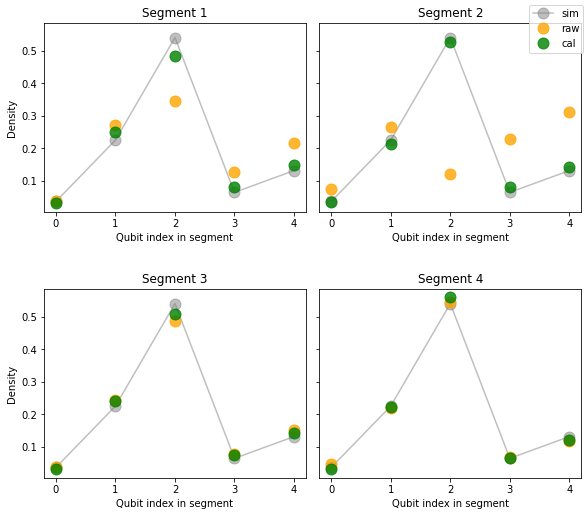We also visualize the mean and variance of results over segments as before.

"""Plot mean density and variance over segments."""
raw_avg = np.average(raw_densities, axis=0)
raw_std = np.std(raw_densities, axis=0, ddof=1)

cal_avg = np.average(cal_densities, axis=0)
cal_std = np.std(cal_densities, axis=0, ddof=1)

plot_density(
plt.gca(),
sim_density,
raw_avg,
cal_avg,
raw_std,
cal_std,
title="Average over segments"
)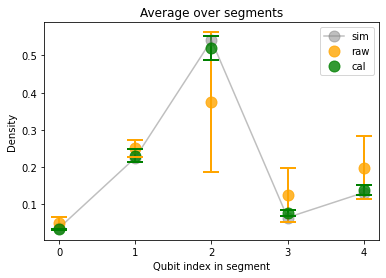Last, we can look at density errors between raw/calibrated results and simulated results.

"""Plot errors of raw vs calibrated results."""
fig, axes = plt.subplots(ncols=2, figsize=(15, 4))

axes.set_title("Error of the mean")
axes.set_ylabel("Density")
axes.set_title("Data standard deviation")

colors = ["orange", "green"]
labels = ["raw", "cal"]

for index, density in enumerate([raw_densities, cal_densities]):
color = colors[index]
label = labels[index]

average_density = np.average(density, axis=0)
sites = list(range(len(average_density)))

error = np.abs(average_density - sim_density)
std_dev = np.std(density, axis=0, ddof=1)

axes.plot(sites, error, color=color, alpha=0.6)
axes.scatter(sites, error, color=color)

axes.plot(sites, std_dev, label=label, color=color, alpha=0.6)
axes.scatter(sites, std_dev, color=color)

for ax in axes:
ax.set_xticks(sites)
ax.set_xlabel("Qubit index in segment")

plt.legend();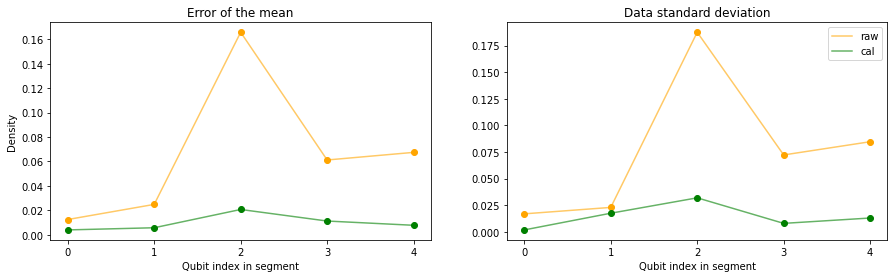[{ "type": "thumb-down", "id": "missingTheInformationINeed", "label":"Missing the information I need" },{ "type": "thumb-down", "id": "tooComplicatedTooManySteps", "label":"Too complicated / too many steps" },{ "type": "thumb-down", "id": "outOfDate", "label":"Out of date" },{ "type": "thumb-down", "id": "samplesCodeIssue", "label":"Samples / code issue" },{ "type": "thumb-down", "id": "otherDown", "label":"Other" }]
[{ "type": "thumb-up", "id": "easyToUnderstand", "label":"Easy to understand" },{ "type": "thumb-up", "id": "solvedMyProblem", "label":"Solved my problem" },{ "type": "thumb-up", "id": "otherUp", "label":"Other" }]